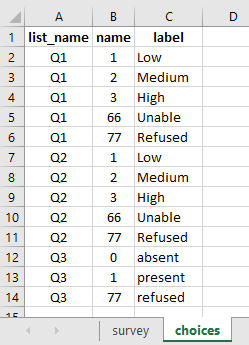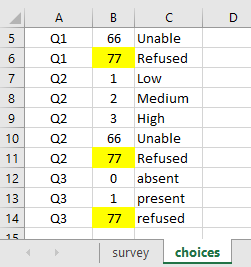# Problems with summation and missing or non acceptable values

Aline_issues.xlsx (10.3 KB) Hello to all,
I really need some help.
I have issues in my summations when missing data, or especially, 66 and 77 values, that for me do not have to be considered in the sum.
I am working on sums for getting scores. Normally, when there is a missing or DNK variable (88, 99, 77) in the sum, the score cannot be calculated. However, I still want to be able to have a sum without considering 77, 88, and 99 … (If a variable has got 77 I take it out from my calculation)

I would like to sum 3 items (A, B, and C).
If A has got a missing value or a 66 (unable to get) or a 77 (refused to have te test) value, I still want to have the sum of B and C (but I do not want the sum to consider 66 or 77 from A).
I tried something with if A=66 then put 0 or leave it as the entered value in my questionnaire.
in attached file an example

@alinephilibert, you could do it as outlined in the image shared below:

#### In the choices tab of your xlsform:#### Reference xlsform:

Aline_issues.xlsx (11.5 KB)

Note: You could use the read_only to block the changes of the result or also use the note question type to display the answer/calculation in a separate response.

1 Like

Dear Kal Lam thank you very very much. as usual you save my ‘statistical life’.
I have parallel questions regarding to the file Aline_issues(2).
1- the solution is
if(\${Q1}>=1 and \${Q1}<=3,\${Q1},‘0’) + if(\${Q2}>=1 and \${Q2}<=3,\${Q2},‘0’) + if(\${Q3}>=0 and \${Q3}<=1,\${Q3},‘0’)
can I write that for not considering 66 and 77? Ido not know what tow rite for missing ‘.’?
if(\${Q1}!=66 and \${Q1}!=77,\${Q1},‘0’) + \${Q2}!=66 and \${Q2}!=77,\${Q1},‘0’) + \${Q1}!=66 and \${Q3}!=77,\${Q3},‘0’)

2-if myQ1 has got values from 1 to 100…how can I do with my 66 and 77? the same as I tried above in 1-?
I was thinking…if(\${Q1}!=66 and \${Q1}!=3,\${Q1},‘0’) + …’)

thank you again and gainIn this case, you could do it as outlined in the image shared below:

#### Reference xlsform:

Aline_issues.xlsx (8.8 KB)

re hello and thank you Kal Lam
Is it possible to have missing answer, a rue missing answer like a empty cell in the output file in excel?
every time I m trying to have an empty response KTB put option_x…otehrwise I can out a 99…just to know.
thank you
Aline

re re hello
my last question
in the solution you put integer in the excel file. if the sum have got decimal?
Thank you againAline

The workaround shared above should solve this issue of managing missing cases through the `if-function`.

That should too work.

Thank you very much.
same story as above Q1, Q2 and Q3. 66 means do not know. Is there a possible calculation to know how many times there was a 66 (from Q1 to Q3 including)
2- is it possible to have an empty cell as an answer (true missing = empty cell) see Aline_issues2 (I put some responses in yellow in choices).
Than you again and again
AlineAline_issues-2.xlsx (11.7 KB)

.

@alinephilibert, you could do this as outlined in the image shared below:

#### In the choices tab of your xlsform:#### Reference xlsform:

Aline_issues.xlsx (11.5 KB)

Hello and thank you very much!!!I am grateful to having you.
one or two precisions…
a-in the attached file If is replace the number 77 by the character refused, is the calculation still okay?
b- I would like to have some response with an empty cell but KTB automatically give the name of the label…see in the excel sheet choice in the yellow cell.

Thank you again for your helpAline_questions.xlsx (11.8 KB)
Aline

Yes! Maybe you will need to pay around for this to be used to using this.

Sorry was not able to understand this.

Thank you very much!!!

1 Like